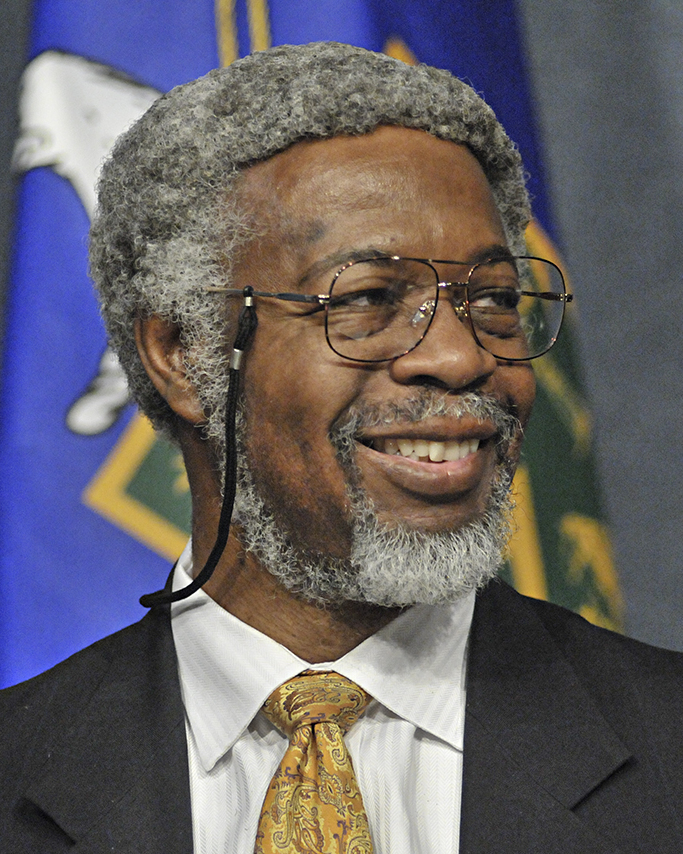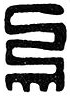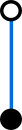# Designing supersymmetry

Studying supersymmetry in physically realistic situations requires a tremendous amount of physical and mathematical sophistication. We’re going to simplify as much as possible: all the way down to zero spatial dimensions!

# Designing supersymmetry

Ursula Whitcher
Mathematical Reviews (AMS)

Mathematicians and physicists both love symmetry, but depending on who you’re talking to, the implications of a simple statement such as “This theory admits a symmetry” can be very different. In this column, I’ll describe one attempt at interdisciplinary translation using an entire rainbow of colors. Our subject is Adinkras. Here is an example:Using Adinkras, we can move from physics questions to algebra questions to combinatorics questions. I’ll sketch ways to think about Adinkras from all three points of view, on the way to providing a formal definition. But to start with, let me introduce you to the inventors of mathematical Adinkras—and the symbols’ namesake.

The physicists Michael Faux and Sylvester James Gates, Jr.—Jim Gates, for short—first described Adinkras in a 2004 paper.Jim Gates (US Department of Energy)Michael Faux

In their introduction, Faux and Gates wrote:

The use of symbols to connote ideas which defy simple verbalization is perhaps one
of the oldest of human traditions. The Asante people of West Africa have long been
accustomed to using simple yet elegant motifs known as Adinkra symbols, to serve
just this purpose. With a nod to this tradition, we christen our graphical symbols as

(Some traditions say the name “Adinkra” derives from the name of an early nineteenth-century ruler of Gyaman, a West African kingdom founded by the Bono people.)

As an example, here are two Adinkra symbols used in early twentieth-century Ghana: Nkyimkyim, the twisted pattern, and Aya, the fern, which can also mean “I am not afraid of you.”Nkyimkyim, the twisted patternAya, the fern

### The physics of supersymmetry

Faux and Gates were motivated by the desire to understand a physical concept known as supersymmetry. This concept arose as a theoretical attempt to organize the vast quantities of information involved in particle physics.

In the Standard Model of particle physics, the fundamental components of the universe are the following types of particles:

• Six leptons (electrons, neutrinos, etc.) and their antiparticles
• Six quarks and their antiparticles.
• Four gauge bosons (photons, gluons, etc.)
• The Higgs boson

Some of these particles make up familiar types of energy and matter. For example, up and down quarks combine to make protons and neutrons, and thus atomic nuclei. Photons are the particles that make up beams of light. Other components of the Standard Model are harder to detect: the IceCube detector at the South Pole hunts for faint flashes of light due to rare neutrino interactions, and confirming the Higgs boson’s existence entailed detailed analysis of particle decay within the particle accelerator known as the Large Hadron Collider.

The fundamental particles in the Standard Model can be divided into two types, bosons and fermions. Bosons transmit fundamental forces—for example, photons carry electromagnetic energy—while we’ve already noted that fermions can combine to make up matter. Other distinctions are more technical. Every boson has an intrinsic integer amount of angular momentum—spin—when measured in units of Planck’s constant $\hbar$, while bosons have half-integer spin ($\frac{1}{2}$, $\frac{3}{2}$, etc.) Furthermore, bosons can cluster: identical bosons can share the same quantum state. In contrast, identical fermions must occupy distinct quantum states, a principle sometimes known as the Pauli exclusion principle.

Supersymmetry is a theoretical physical symmetry that exchanges bosons and fermions. In particular, in a supersymmetric theory, every type of boson must have a fermion partner, and vice versa.“Where is my superpartner?”

Despite years of searching, experimental physicists have not found evidence of superpartners for any of the particles in the Standard Model. That doesn’t mean understanding supersymmetry isn’t useful! After all, a real-world tennis ball’s path will deviate from a perfect parabola due to air resistance, but parabolas are not meaningless. Indeed, the equations underlying quantum supersymmetry have proved useful in understanding optical and condensed-matter systems at larger scales.

### Focusing on time

Studying supersymmetry in physically realistic situations requires a tremendous amount of physical and mathematical sophistication. We’re going to simplify as much as possible: all the way down to zero spatial dimensions! In other words, from now on we’ll assume that all of our bosons and fermions live on a single point.

In physics at the scales where human beings usually operate, once you’ve restricted to a single point, nothing much can happen. But in quantum physics, particles can have intrinsic properties without obviously moving. For example, an electron can act like a single point with angular momentum, but a single point doesn’t have anywhere to spin. We’ll represent the intrinsic information in our model mathematically by writing our bosons and fermions as functions of a time parameter $t$. In physicist’s language, this is a one-dimensional theory: that one dimension is time.

Let’s suppose we have $m$ fundamental bosons, $\{\phi_1, \dots, \phi_m\}$, and $m$ fundamental fermions, $\{\psi_1, \dots, \psi_m\}$. We also assume that we have $N$ supersymmetry operators $\{Q_1, \dots, Q_N\}$. Each supersymmetry operator transforms bosons to fermions, and vice versa. We write this in a notation similar to function notation. For example, $Q_1 \phi_2$ should be a fermion (perhaps one of our fundamental fermions, or perhaps a transformation of a fundamental fermion, such as $-\psi_3$).

In quantum physics, the order in which you do things matters: for example, the outcome of two measurements may depend on which one you try first. That means that $Q_I Q_J$ and $Q_J Q_I$ could be different. We can measure this using the anticommutator, which we write with curly brackets:

$\{A, B\} = AB + BA.$

With this notation in hand, we’re ready to describe the rules that the supersymmetry operators have to follow. In order to do so, we’ll have to use a little bit of calculus notation, namely, the time derivative $\frac{d}{dt}$. If this notation is unfamiliar to you, don’t worry. You can think of $\frac{d}{dt}$ as telling you something about the way a boson or fermion changes over a small period of time. But we’ll replace all our derivatives by diagrams when we start building Adinkras!

Here are the two rules for supersymmetry operators.

• If $I \neq J$, then
$\{Q_I, Q_J\} = 0.$
In other words, for $I \neq J$, $Q_I Q_J = -Q_J Q_I$: if you apply two different supersymmetry operators in two different orders, the results will be opposites.

• Each supersymmetry operator $Q_I$ satisfies
$\{Q_I, Q_I\} = 2 i \frac{d}{dt}.$
Here $i$ is the square root of $-1$. (Physicists have standard rules for translating quantum quantities involving complex numbers into real-world observable measurements.) We can simplify this rule to $Q_I Q_I = i \frac{d}{dt}$.

We’re interested in understanding how many genuinely different solutions to the supersymmetry operator rules we can find. Even for small examples, there may be multiple possibilities. Each one is called a representation of the supersymmetry algebra. For example, suppose we have one fundamental boson $\phi_1$, one fundamental fermion $\psi_1$, and one supersymmetry operator $Q_1$. We need to specify $Q_1 \phi_1$ and $Q_2 \psi_2$. One possibility is:

$$Q_1 \phi_1 = \psi_1$$

$$Q_1 \psi_1 = i \frac{d}{dt} \phi_1.$$

Another possibility is:

$$Q_1 \phi_1 = \frac{d}{dt} \psi_1$$

$$Q_1 \psi_1 = i \phi_1.$$

You can check that in either case, $Q_1 Q_1 \phi_1 = i \frac{d}{dt} \phi_1$ and $Q_1 Q_1 \psi_1 = i \frac{d}{dt} \psi_1$.

### Using combinatorics

To organize our possible supersymmetry operator representations, we use graphs. These are graphs in the sense of graph theory, with vertices (dots) connected by edges (line segments). We use $m$ open vertices and $m$ closed vertices. Each open vertex represents a fundamental boson $\phi_j$ together with its derivatives and constant multiples; each closed vertex represents a fundamental fermion together with its derivatives and multiples. We pick $N$ possible edge colors, one for each of the $N$ supersymmetry operators. We think of acting on a boson or fermion by a supersymmetry operator as traveling along the edge of the appropriate color. Since we can act on any boson or fermion by any of the operators, we need an edge of each possible color attached to every boson vertex and every fermion vertex. Here is an example of the resulting structure.This example has a property we haven’t discussed yet, corresponding to the supersymmetry algebra rule $Q_I Q_J = -Q_J Q_I$. Applying two different supersymmetry operators in different orders yields the same result, up to a sign change. That means that if we pick a vertex and a pair of edge colors, it shouldn’t matter which edge we follow first: for example, blue then green should have the same result as green then blue.

We can summarize the requirements we’ve described so far using the notion of a chromotopology. A chromotopology is a finite simple graph (that is, it has a finite number of vertices and edges, and none of the edges starts and ends in the same place) with the following properties:

• Bipartite (there are two types of vertices, and each edge has one endpoint of each type)
• $N$-regular with a consistent edge coloring (every edge is colored one of $N$ colors, and every vertex has $N$ incident edges, one of each color)
• Quadrilateral (every simple cycle with two colors is of length 4).

The quadrilateral property is a different way of describing the requirement that following a pair of edge colors in either order has the same result: instead of considering two possible paths away from the same vertex, we notice that following one path away from a vertex and the other path back again brings us back to the start (so blue-green-blue-green will return us to the vertex where we started).

To make an Adinkra, we start with a chromotopology and add two additional structures. The first is a height assignment. To make a height assignment, we place our bosons and fermions on different levels, and require that every time we follow an edge, we go up or down one level. For example, when $N=1$, there are two possible height assignments, one with the boson on the lowest level and one with the fermion on the lowest level. You might notice that the example Adinkra and chromotopology we’ve already seen are drawn with consistent height assignments.$N=1$ height assignment, boson on the lowest level$N=1$ height assignment, fermion on the lowest level

In the conversion between Adinkras and supersymmetry representations, the height assignment tells us when to take derivatives. We use the convention that going up a level does not use a time derivative, but going back down does. Thus, in the one-dimensional example, our choice of height specifies whether we take a derivative when going from fermion to boson or from boson to fermion.

Our final structure is an odd dashing. We know that in a chromotopology, picking a vertex and a pair of colors gives us a 4-cycle of edges that will bring us back where we started. To make an odd dashing, for each of these 4-cycles, we dash an odd number of edges—either just one, or three of the four. For example, here is a possible dashing of our example $N=3$ chromotopology—making it into an Adinkra.Here is a different odd dashing of the same chromotopology—and thus a different Adinkra!The dashings tell us where to place minus signs in our supersymmetry representations. Because there are an odd number of dashed edges in each two-color 4-cycle, when we flip the order in which we follow a pair of colors, we will also change the number of dashed edges we encounter. This guarantees that the minus sign condition in the supersymmetry algebra rule $Q_I Q_J = -Q_J Q_I$ will be satisfied.

Adinkras help us generate a multitude of supersymmetry algebra representations. They also give us new ways to visualize relationships between distinct representations: for example, we can classify supersymmetry algebra representations that correspond to distinct dashings of the same chromotopology and height assignment, or move from representation to representation by raising and lowering vertices to different levels. The result is a powerful tool for exploration and discovery, in both physics and mathematics.

### Further exploration

Charles F. Doran, Michael G. Faux, S. James Gates, Jr., Tristan Hübsch, Kevin M. Iga, Gregory D. Landweber, and Robert L. Miller, Codes and supersymmetry in one dimension.
Adv. Theor. Math. Phys. Vol. 15, No. 6 (2011), 1909-1970
A classification of chromotopologies using error-correcting codes.
Michael Faux and S. James Gates, Jr., Adinkras: A graphical technology for supersymmetric representation theory.
Phys. Rev. D (3) Vol. 71 (2005), 065002